Related Articles

# MongoDB – Multiply Operator (\$mul)

• Last Updated : 10 May, 2020

MongoDB provides different types of field update operators to update the values of the fields of the documents and \$mul operator is one of them. This operator is used to multiply the value of the field by a number.

• \$mul operator only updates those fields whose value are of numeric type like int, float, etc.
• If the specified field is not present in the document, then this operator will add that field in the document and assign the value of that field to zero of the same numeric type as the multiplier.
• This operator is an atomic operation within a single document.
• In this operator, multiplication with values of mixed numeric types like 32-bit integer, 64-bit integer, float, etc., may result in conversion of numeric type. The following rules are applied in the multiplication with the values of mixed numeric types:
32-bit Integer64-bit IntegerFloat
32-bit Integer32-bit or 64-bit Integer64-bit IntegerFloat
64-bit Integer64-bit Integer64-bit IntegerFloat
FloatFloatFloatFloat

\$mul operator can also work with embedded/nested documents or arrays. You can use this operator in methods like update(), findAndModify(), etc., according to your requirements.

Syntax:

`{ \$mul: { <field1>: <number1>, <field2>: <number2>, ... } }`

Here, `<field>` can specify with dot notation in embedded/nested documents.

In the following examples, we are working with:

Database: Fruits
Collection: Details
Document: two documents that contain the details of the fruits in the form of field-value pairs.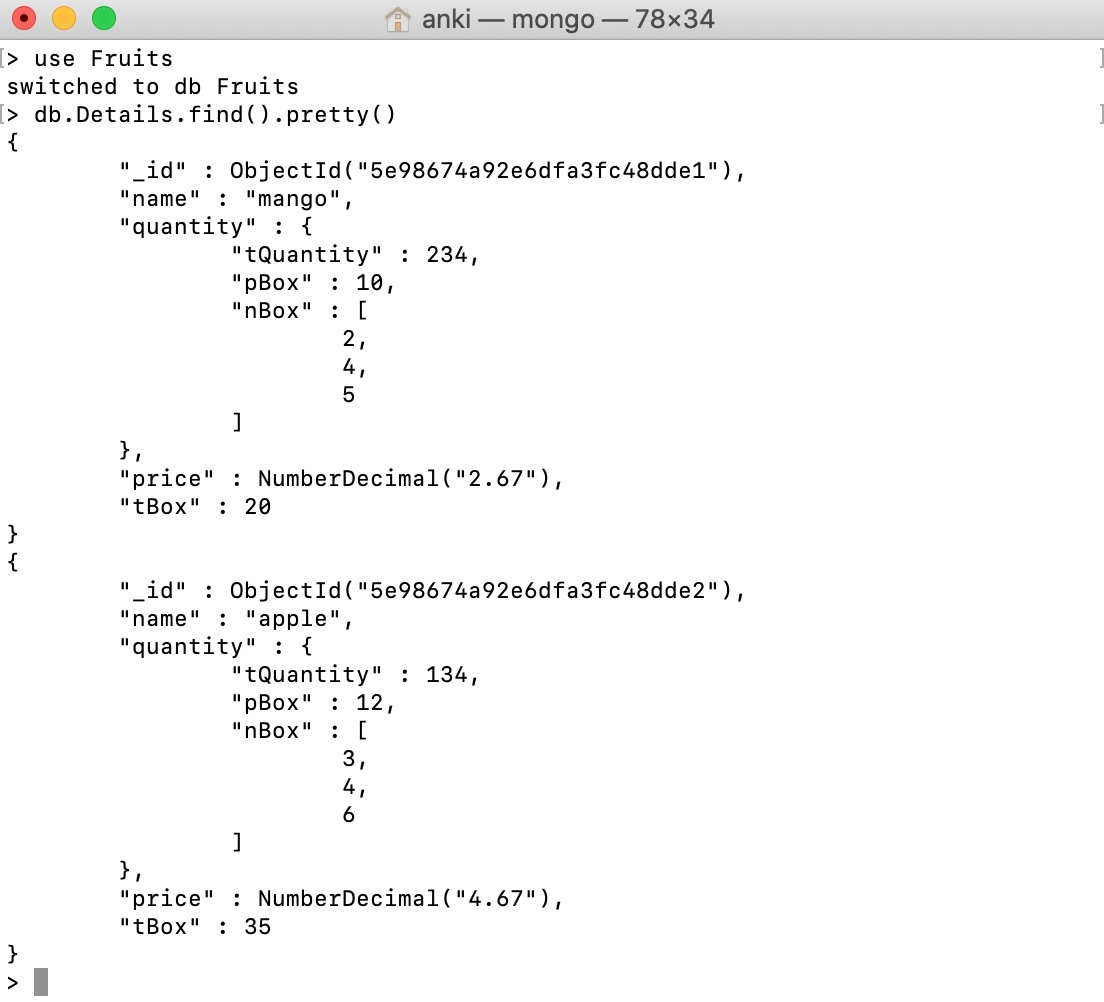## Multiplying the value of a field:

In this example, we are multiplying the value of price field by 2.10 in the document who matches the specified condition, i.e., name = mango.

 `db.Details.update({name: ``"mango"``}, {\$mul: {price: NumberDecimal(``"2.10"``)}})`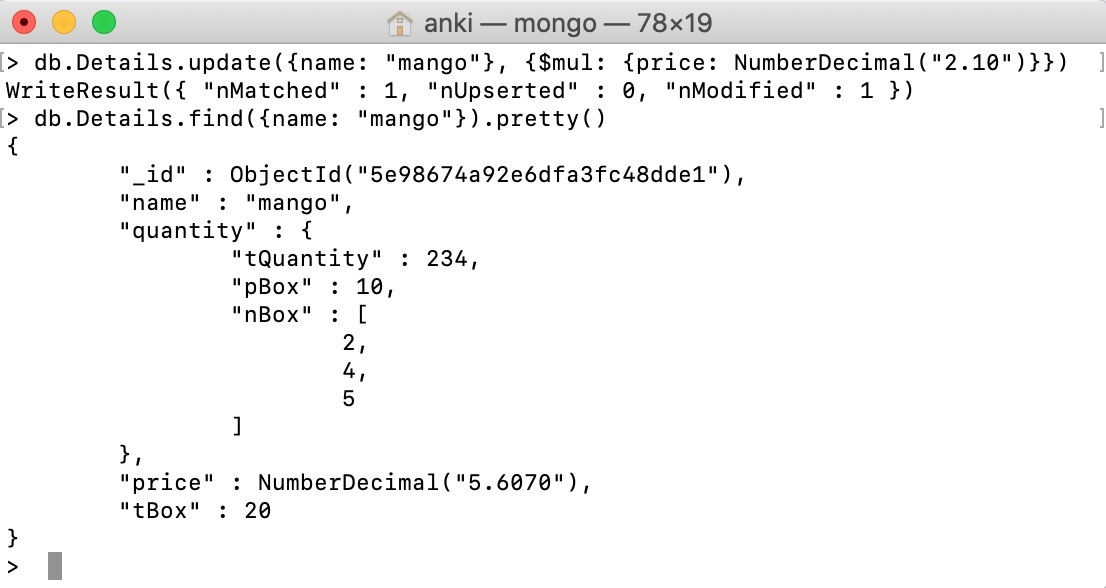## Multiplying the value of a field in the embedded/nested document:

In this example, we are multiplying the value of quantity.tQuantity field by 3 in the document who matches the specified condition, i.e., name = mango.

 `db.Details.update({name: ``"mango"``}, {\$mul: {``"quantity.tQuantity"``: ``3``}})`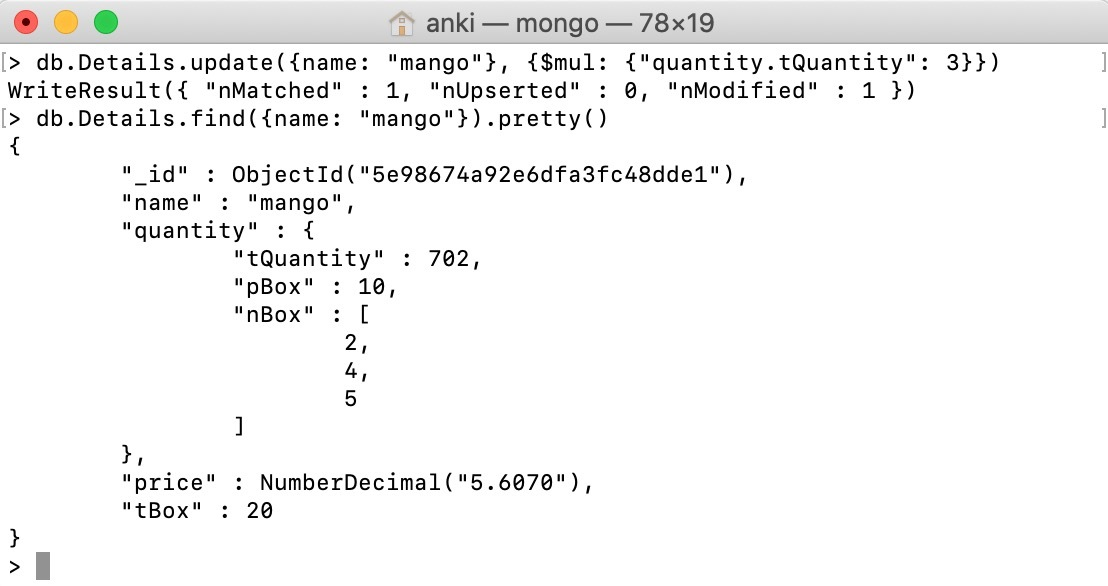## Using` \$mul `operator to a non-existing field:

In this example, we are applying \$mul operator to a non- existing field in the document who matches the specified condition, i.e., name = apple.

 `db.Details.update({name: ``"apple"``}, {\$mul: {``"batchNumber"``:NumberInt(``230``)}})`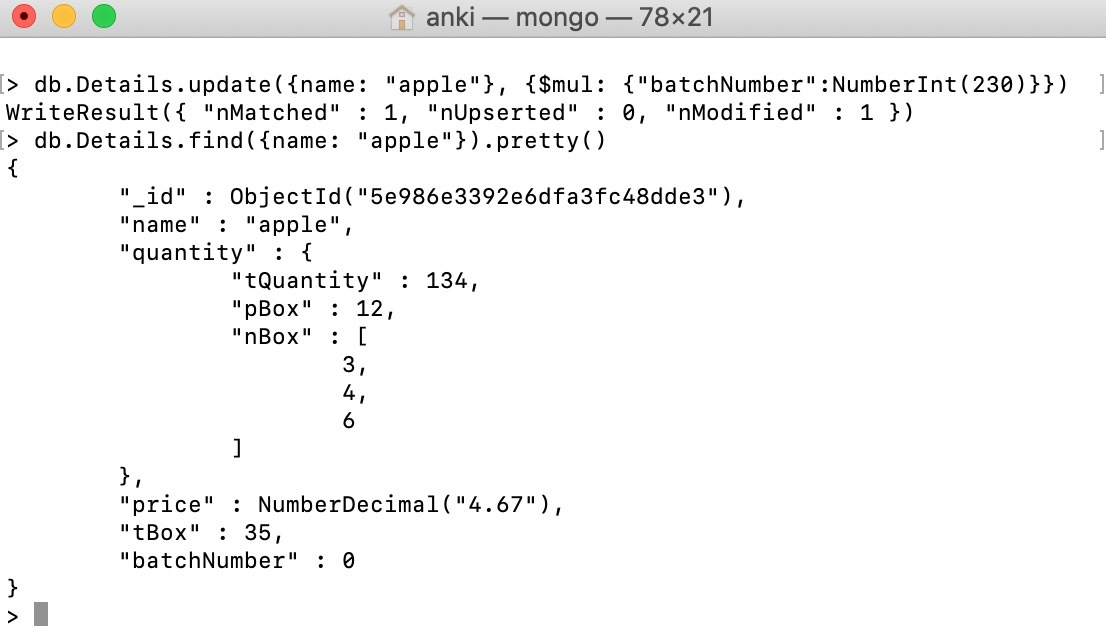## Using `\$mul `operator to multiply mixed numeric type:

In this example, we are multiplying the value(float type) of price field in the document who matches the specified condition, i.e., name = apple.

 `db.Details.update({name: ``"apple"``}, {\$mul: {price: NumberDecimal(``5``)}})`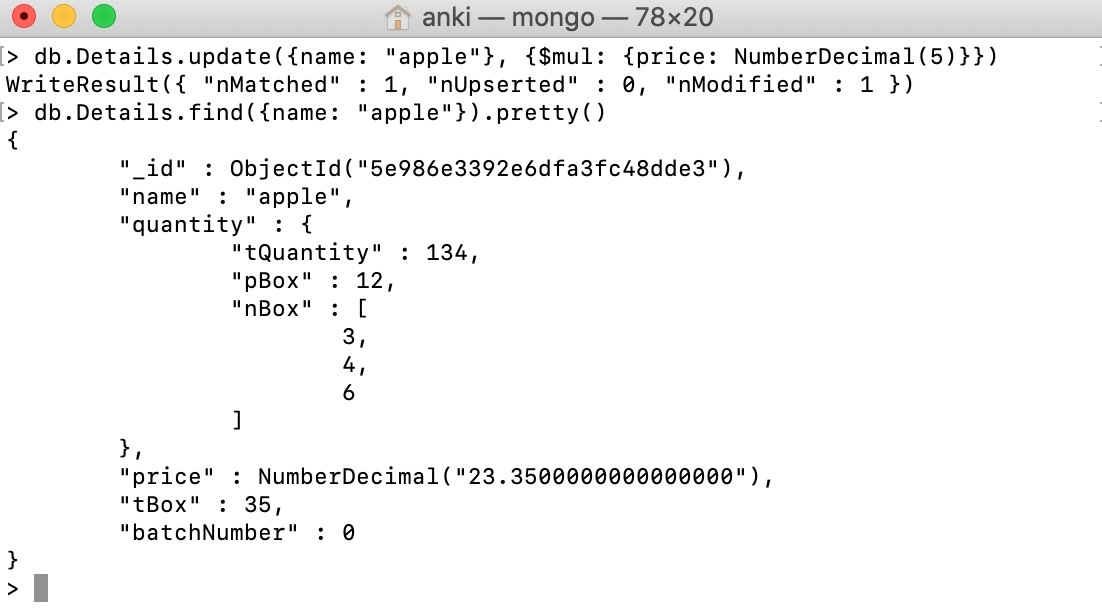My Personal Notes arrow_drop_up